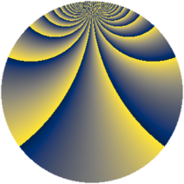# Properties

 Label 1805.2.biLevel $1805$ Weight $2$ Character orbit 1805.bi Rep. character $\chi_{1805}(2,\cdot)$ Character field $\Q(\zeta_{684})$ Dimension $40608$ Sturm bound $380$

# Related objects

## Defining parameters

 Level: $$N$$ $$=$$ $$1805 = 5 \cdot 19^{2}$$ Weight: $$k$$ $$=$$ $$2$$ Character orbit: $$[\chi]$$ $$=$$ 1805.bi (of order $$684$$ and degree $$216$$) Character conductor: $$\operatorname{cond}(\chi)$$ $$=$$ $$1805$$ Character field: $$\Q(\zeta_{684})$$ Sturm bound: $$380$$

## Dimensions

The following table gives the dimensions of various subspaces of $$M_{2}(1805, [\chi])$$.

Total New Old
Modular forms 41472 41472 0
Cusp forms 40608 40608 0
Eisenstein series 864 864 0

## Trace form

 $$40608 q - 216 q^{2} - 216 q^{3} - 216 q^{5} - 444 q^{6} - 222 q^{7} - 210 q^{8} + O(q^{10})$$ $$40608 q - 216 q^{2} - 216 q^{3} - 216 q^{5} - 444 q^{6} - 222 q^{7} - 210 q^{8} - 216 q^{10} - 444 q^{11} - 210 q^{12} - 216 q^{13} - 234 q^{15} - 468 q^{16} - 198 q^{17} - 228 q^{18} - 144 q^{20} - 480 q^{21} - 204 q^{22} - 228 q^{23} - 240 q^{25} - 456 q^{26} - 210 q^{27} - 228 q^{28} - 222 q^{30} - 420 q^{31} - 246 q^{32} - 90 q^{33} - 198 q^{35} - 480 q^{36} - 228 q^{37} - 282 q^{38} - 282 q^{40} - 468 q^{41} - 252 q^{42} - 48 q^{43} - 240 q^{45} - 420 q^{46} - 204 q^{47} - 288 q^{48} - 354 q^{50} - 360 q^{51} - 228 q^{52} - 198 q^{53} - 246 q^{55} - 456 q^{56} - 162 q^{57} - 348 q^{58} - 312 q^{60} - 408 q^{61} - 288 q^{62} - 102 q^{63} - 300 q^{65} - 528 q^{66} - 336 q^{67} - 108 q^{68} - 264 q^{70} - 432 q^{71} - 276 q^{72} - 234 q^{73} - 228 q^{75} - 516 q^{76} - 288 q^{77} - 90 q^{78} - 168 q^{80} - 336 q^{81} - 288 q^{82} - 204 q^{83} - 192 q^{85} - 2328 q^{86} - 222 q^{87} - 30 q^{88} + 42 q^{90} - 204 q^{91} - 156 q^{92} - 138 q^{93} + 252 q^{95} - 360 q^{96} - 156 q^{97} - 138 q^{98} + O(q^{100})$$

## Decomposition of $$S_{2}^{\mathrm{new}}(1805, [\chi])$$ into newform subspaces

The newforms in this space have not yet been added to the LMFDB.